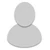## FANDOM

217 個頁面

•   正在載入編輯器
• ？？？？？
不要丟一個外部連結然後都不解釋，用WIN7的我會怕w

正在載入編輯器
• 這是在一個網站看到的

The Useless Web

(沒用的網站)

正在載入編輯器
• :thinking: 還是不要亂點好了，我很怕（

正在載入編輯器
• 好吧我還是點了 我大概知道這東西怎麼弄了 只是我還是做不出來
誰這麼無聊啊

正在載入編輯器
• 可以用檢視網頁原始碼阿

正在載入編輯器
•  ,
_,,)\.~,,._
(()`  ``)\))),,_
|     \ ((\)))),,_          ____
|6`   |
((\())) "-.____.-"    `-.-,
|    .'\    ))))'                  \)))
|   |   `.
((((
\, _)     \/                          |))))
`'        |                          (((((
\                  |       ))))))
`|    |           ,\     /((((((
|   / `-.______.<  \   |  )))))
|   |  /         `. \  \  ((((
|  / \ |           `.\  | (((
\  | | |             )| |  ))
| | | |             || |  '

正在載入編輯器
• 還有這個加強版

正在載入編輯器
• 好喔

正在載入編輯器
•正在載入編輯器